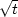Next: Proof by way of Up: TIME-FREQUENCY RESOLUTION Previous: Gabor's proof of the

## My rise-time proof of the uncertainty principle

In FGDP I came up with a proof of the uncertainty principle that is appropriate for causal functions. That proof is included directly below, but I recommend that the beginning reader skip over it, as it is somewhat lengthy. I include it because this book is oriented toward causal functions, the proof is not well known, and I have improved it since FGDP.

A similar and possibly more basic concept than the product of time and frequency spreads is the relationship between spectral bandwidth and the rise time'' of a system-response function. The rise timeof a system response is defined as follows: when we kick a physical system with an impulse function, it usually responds rapidly, rising to some maximum level, and then dropping off more slowly toward zero. The quantitative value of the rise time is generally, and somewhat arbitrarily, taken to be the span between the time of excitation and the time at which the system response is more than halfway up to its maximum.

Tightness" (nearness to equality) in the inequality (1) is associated with minimum phase. Slackness" (remoteness from equality) in the (1) would occur if a filter with an additional all-pass component were used. Slackness could also be caused by a decay time that is more rapid than the rise time, or by other combinations of rises and falls, such as random combinations. Minimum-phase systems generally respond rapidly compared to the rate at which they later decay. Focusing our attention on such systems, we can now seek to derive the inequality (1) applied to rise time and bandwidth.

The first step is to choose a definition for rise time. I have found a tractable definition of rise time to be(3)
where b(t) is the response function under consideration. Equation (3) definesby the first negative moment. Since this is unfamiliar, consider two examples. Taking b(t) to be a step function, recognize that the numerator integral diverges, giving the desiredrise time. As a further example, take b(t)2 to grow linearly from zero to t0 and then vanish. Then the rise timeis t0/2, again reasonable. It is curious that b(t) could grow as,which rises with infinite slope at t=0, and not causeto be pushed to zero.Next: Proof by way of Up: TIME-FREQUENCY RESOLUTION Previous: Gabor's proof of the
Stanford Exploration Project
10/21/1998